Chapter 1 Class 12 Relation and Functions

Class 12
Important Questions for exams Class 12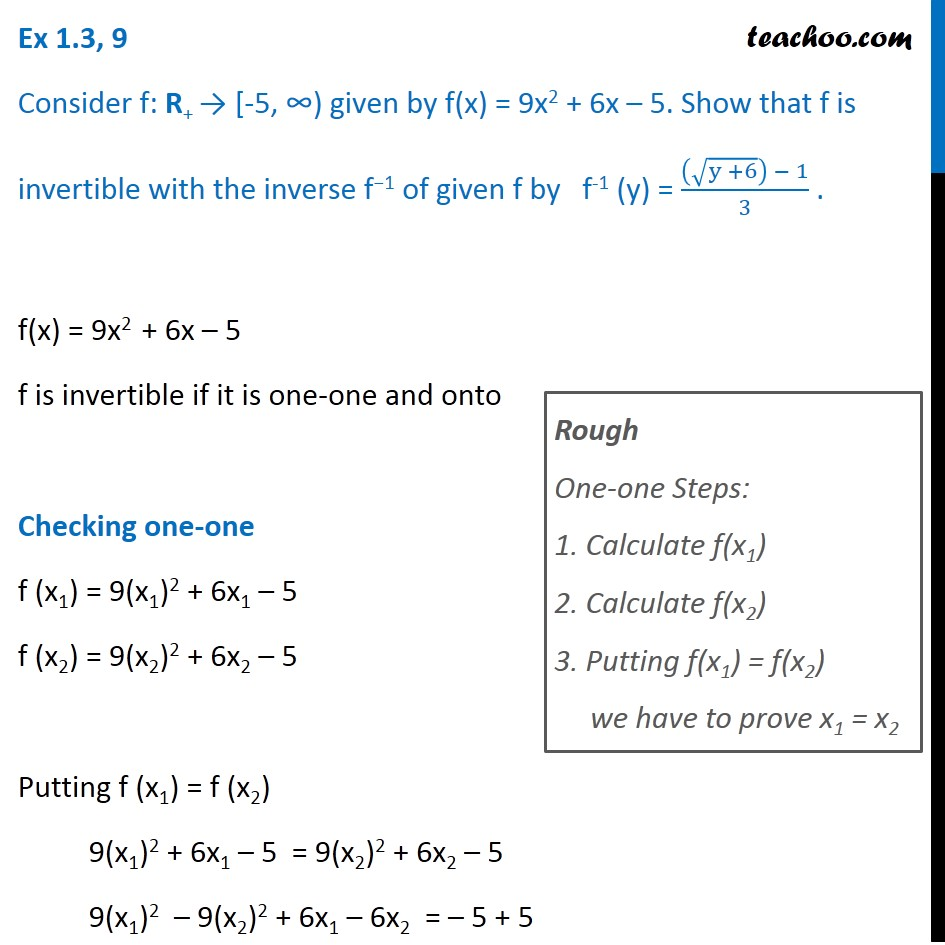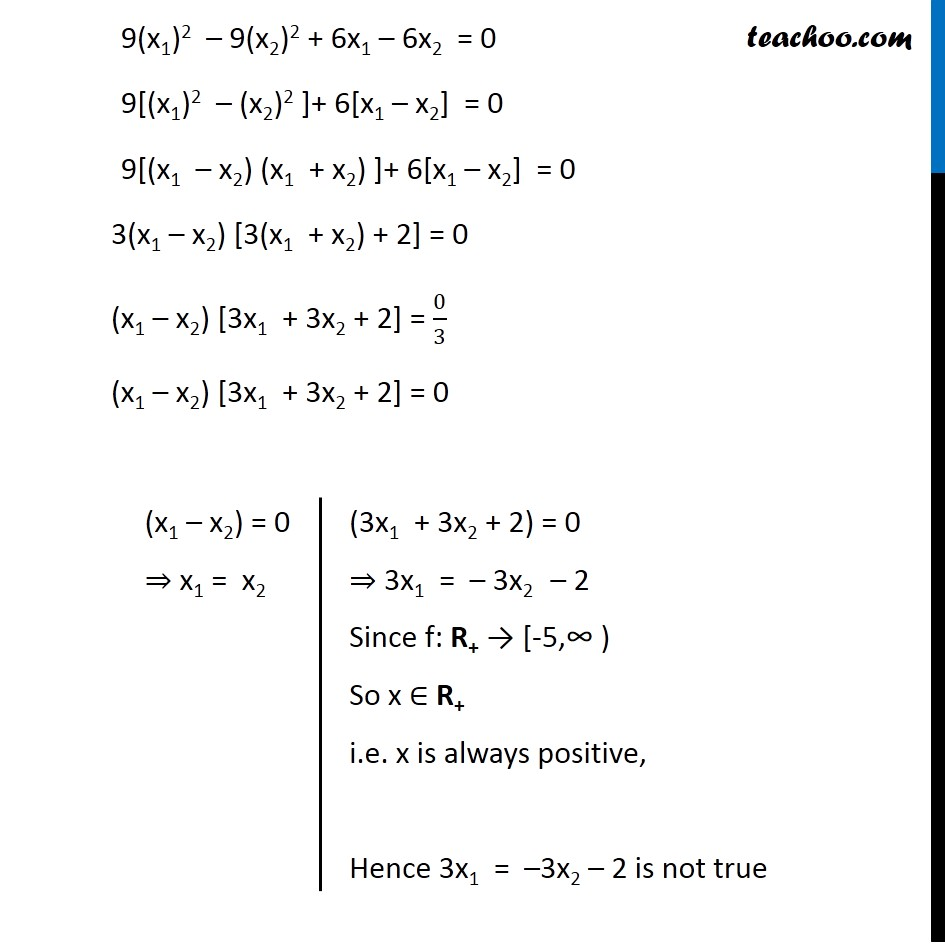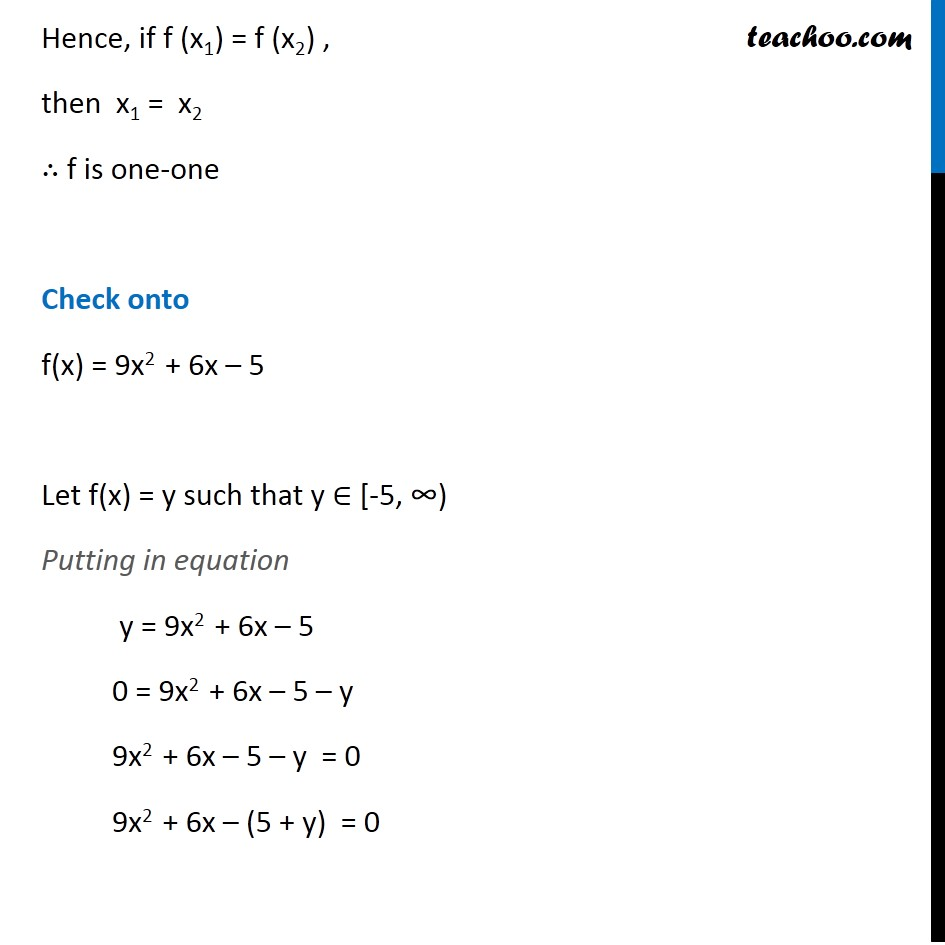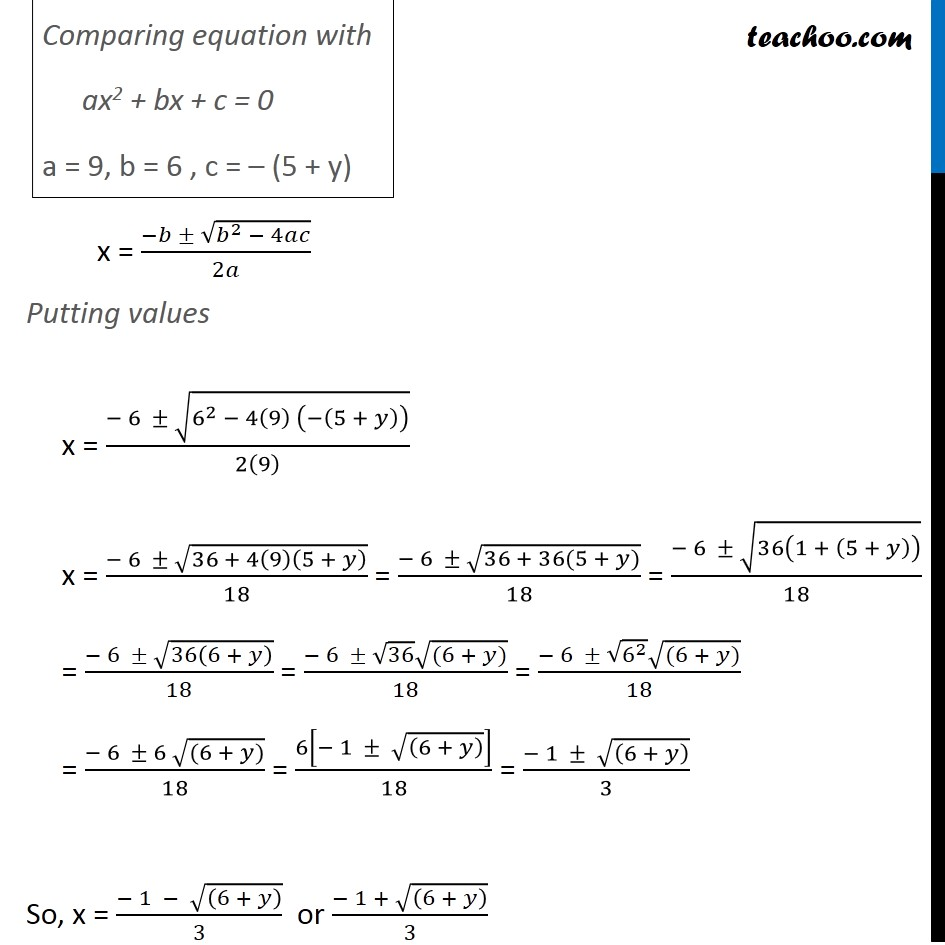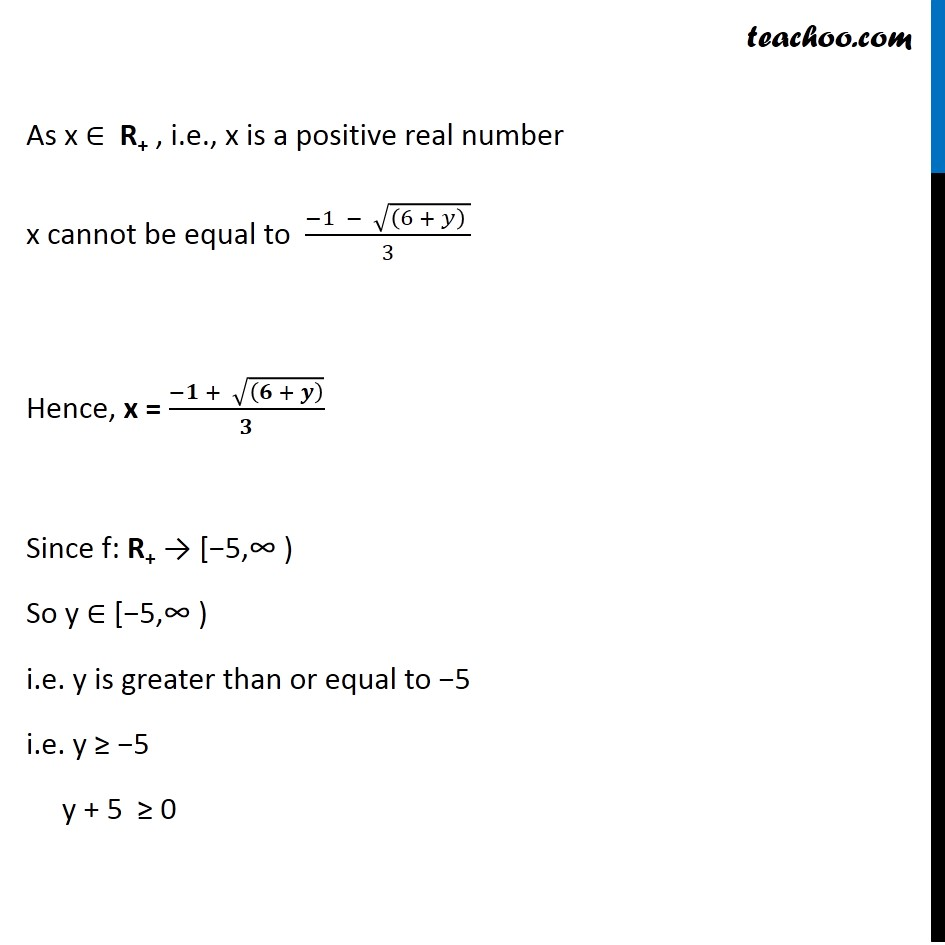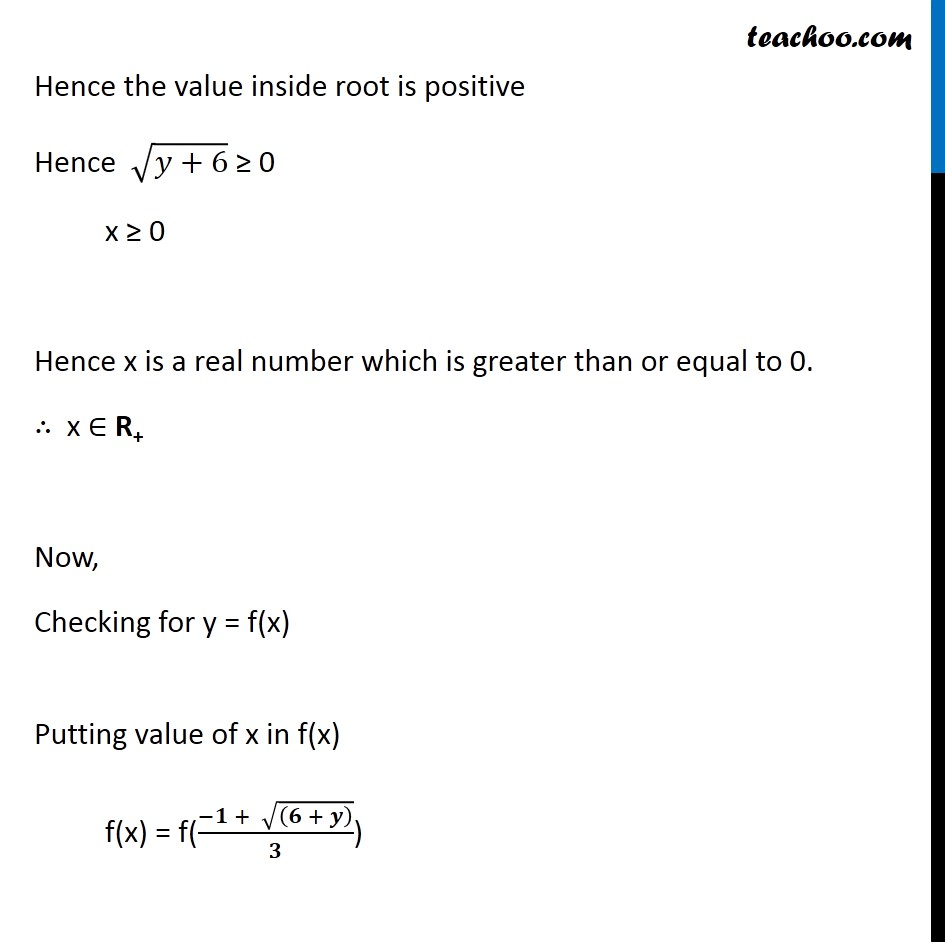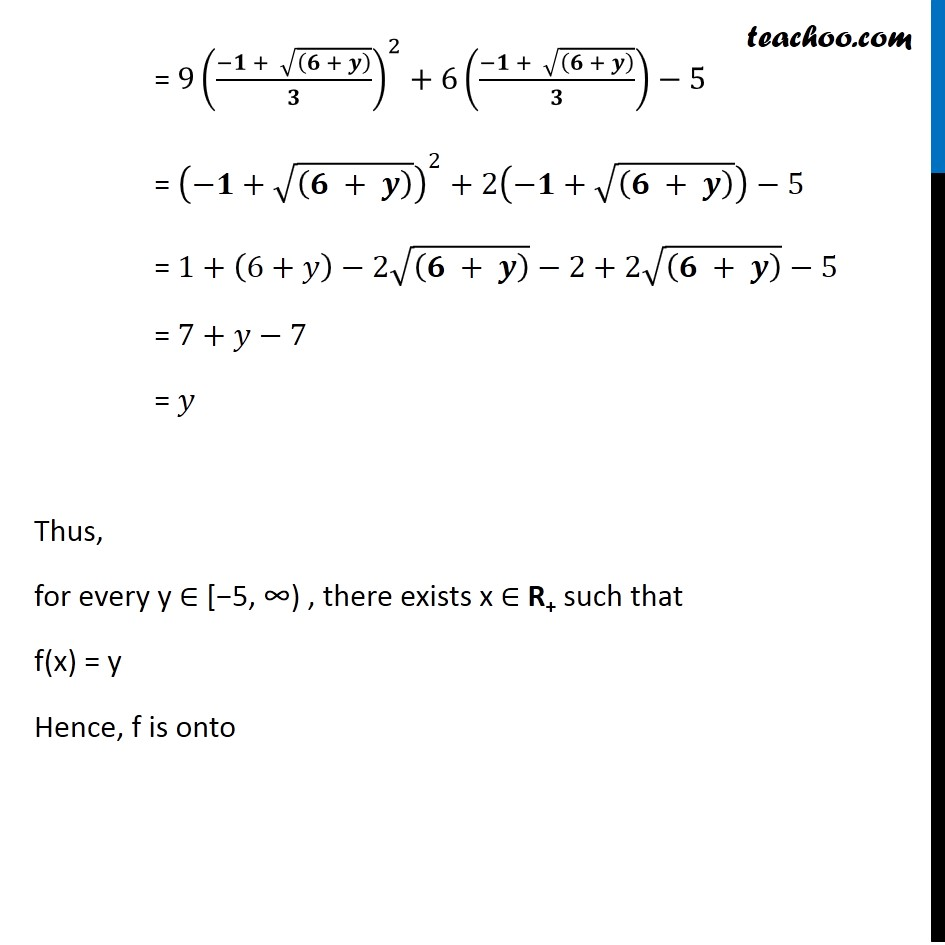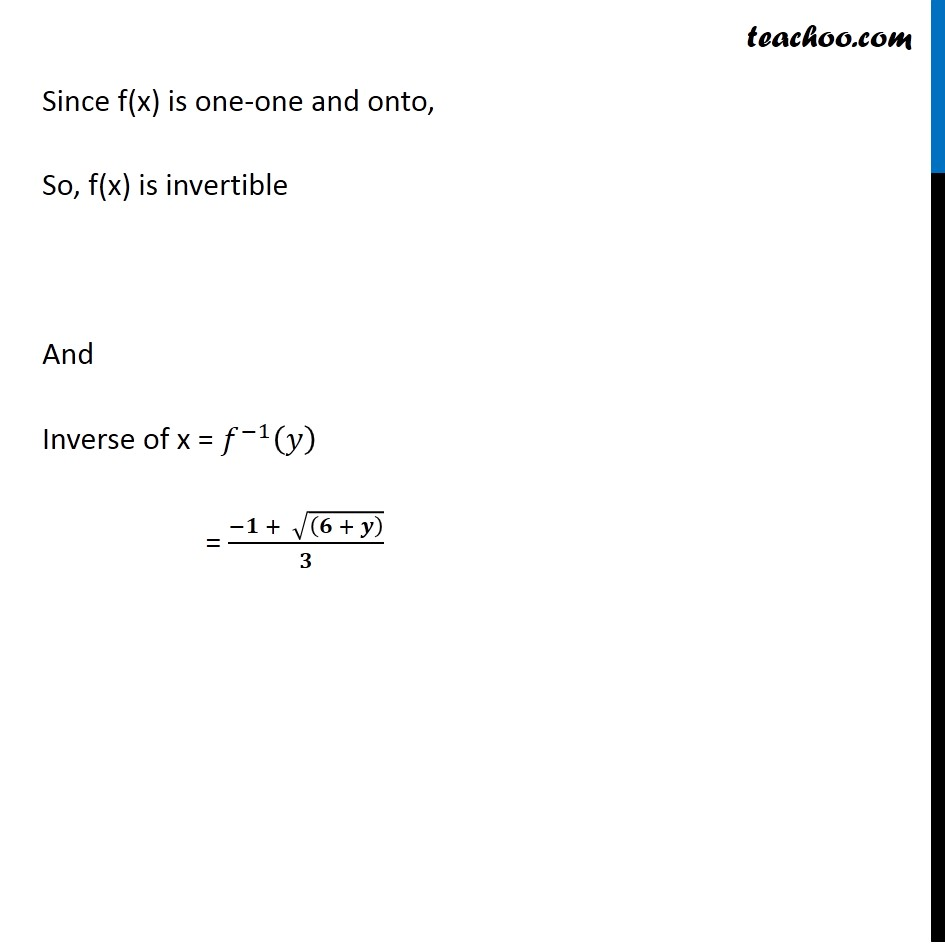Introducing your new favourite teacher - Teachoo Black, at only ₹83 per month

### Transcript

Ex 1.3, 9 Consider f: R+ → [-5, ∞) given by f(x) = 9x2 + 6x – 5. Show that f is invertible with the inverse f−1 of given f by f-1 (y) = ((√(y +6)) − 1)/3 . f(x) = 9x2 + 6x – 5 f is invertible if it is one-one and onto Checking one-one f (x1) = 9(x1)2 + 6x1 – 5 f (x2) = 9(x2)2 + 6x2 – 5 Putting f (x1) = f (x2) 9(x1)2 + 6x1 – 5 = 9(x2)2 + 6x2 – 5 9(x1)2 – 9(x2)2 + 6x1 – 6x2 = – 5 + 5 Rough One-one Steps: 1. Calculate f(x1) 2. Calculate f(x2) 3. Putting f(x1) = f(x2) we have to prove x1 = x2 9(x1)2 – 9(x2)2 + 6x1 – 6x2 = 0 9[(x1)2 – (x2)2 ]+ 6[x1 – x2] = 0 9[(x1 – x2) (x1 + x2) ]+ 6[x1 – x2] = 0 3(x1 – x2) [3(x1 + x2) + 2] = 0 (x1 – x2) [3x1 + 3x2 + 2] = 0/3 (x1 – x2) [3x1 + 3x2 + 2] = 0 (x1 – x2) = 0 ⇒ x1 = x2 (3x1 + 3x2 + 2) = 0 ⇒ 3x1 = – 3x2 – 2 Since f: R+ → [-5,∞ ) So x ∈ R+ i.e. x is always positive, Hence 3x1 = –3x2 – 2 is not true Hence, if f (x1) = f (x2) , then x1 = x2 ∴ f is one-one Check onto f(x) = 9x2 + 6x – 5 Let f(x) = y such that y ∈ [-5, ∞) Putting in equation y = 9x2 + 6x – 5 0 = 9x2 + 6x – 5 – y 9x2 + 6x – 5 – y = 0 9x2 + 6x – (5 + y) = 0 Comparing equation with ax2 + bx + c = 0 a = 9, b = 6 , c = – (5 + y) x = (−𝑏 ± √(𝑏^2 − 4𝑎𝑐))/2𝑎 Putting values x = (− 6 ± √(6^2 − 4(9) (−(5 + 𝑦)) ))/2(9) x = (− 6 ± √(36 + 4(9)(5 + 𝑦) ))/18 = (− 6 ± √(36 + 36(5 + 𝑦)))/18 = (− 6 ± √(36(1 + (5 + 𝑦)) ))/18 = (− 6 ± √(36(6 + 𝑦) ))/18 = (− 6 ± √36 √((6 + 𝑦)))/18 = (− 6 ± √(6^2 ) √((6 + 𝑦)))/18 = (− 6 ± 6 √((6 + 𝑦)))/18 = 6[− 1 ± √((6 + 𝑦) )]/18 = (− 1 ± √((6 + 𝑦) ))/3 So, x = (− 1 − √((6 + 𝑦) ))/3 or (− 1 + √((6 + 𝑦) ))/3 As x ∈ R+ , i.e., x is a positive real number x cannot be equal to (−1 − √((6 + 𝑦) ))/3 Hence, x = (−𝟏 + √((𝟔 + 𝒚) ))/𝟑 Since f: R+ → [−5,∞ ) So y ∈ [−5,∞ ) i.e. y is greater than or equal to −5 i.e. y ≥ −5 y + 5 ≥ 0 Hence the value inside root is positive Hence √(𝑦+6) ≥ 0 x ≥ 0 Hence x is a real number which is greater than or equal to 0. ∴ x ∈ R+ Now, Checking for y = f(x) Putting value of x in f(x) f(x) = f((−𝟏 + √((𝟔 + 𝒚) ))/𝟑) = 〖9((−𝟏 + √((𝟔 + 𝒚) ))/𝟑)〗^2+6((−𝟏 + √((𝟔 + 𝒚) ))/𝟑)−5 = (−𝟏+√((𝟔 + 𝒚) ))^2+2(−𝟏+√((𝟔 + 𝒚) ))−5 = 1+(6+𝑦)−2√((𝟔 + 𝒚) )−2+2√((𝟔 + 𝒚) )−5 = 7+𝑦−7 = 𝑦 Thus, for every y ∈ [−5, ∞) , there exists x ∈ R+ such that f(x) = y Hence, f is onto Since f(x) is one-one and onto, So, f(x) is invertible And Inverse of x = 𝑓^(−1) (𝑦) = (−𝟏 + √((𝟔 + 𝒚) ))/𝟑